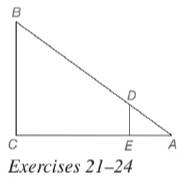Chapter 5.2, Problem 22EElementary Geometry For College St...

7th Edition
Alexander + 2 others
ISBN: 9781337614085

Solutions

Chapter
SectionElementary Geometry For College St...

7th Edition
Alexander + 2 others
ISBN: 9781337614085
Textbook Problem

In Exercises 21 to 24, △ A D E ∼ △ A B C . Given: D E = 5 , A D = 8 , D B = B C Find: A B (HINT: Find D B first.)To determine

To calculate:

The value of the side AB.

Explanation

Given:

It is given that ΔADEABC.

Definition Used:

Similarity between Two polygons:

Two polygons are similar if and only if when all pairs of corresponding angles are congruent and all pairs of corresponding sides are proportional.

If C is a point of XY¯ and XCY, then XC+CY=XY.

Calculation:

According to the definition of similar polygon, ACAE=BCDE=ABAD.

Therefore,

Still sussing out bartleby?

Check out a sample textbook solution.

See a sample solution

The Solution to Your Study Problems

Bartleby provides explanations to thousands of textbook problems written by our experts, many with advanced degrees!

Get Started

Find the mean for the scores in the following frequency distribution table: X f 6 1 5 4 4 2 3 2 2 1

Essentials of Statistics for The Behavioral Sciences (MindTap Course List)

34. If , find the following.

Mathematical Applications for the Management, Life, and Social Sciences

Fill in the blanks. 8. a. The general form of an equation of a line is_______.

Applied Calculus for the Managerial, Life, and Social Sciences: A Brief Approach

ex(ex)2 = a) ex3 b) e3x c) 3ex d) e4x

Study Guide for Stewart's Single Variable Calculus: Early Transcendentals, 8th